A particle of mass m, initially at rest, is acted upon by a variable force F for a brief interval of time T. It begins to move with a velocity u after the force stops acting. F is shown in the graph as a function of time. The curve is an ellipse.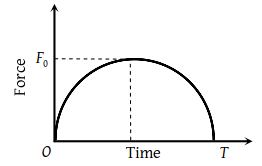(1) $u=\frac{\pi {F}_{0}^{2}}{2m}$

(2) $u=\frac{\pi {T}^{2}}{8m}$

(3) $u=\frac{\pi {F}_{0}T}{4m}$

(4) $u=\frac{{F}_{0}T}{2m}$

Concept Questions :-

Newton laws
High Yielding Test Series + Question Bank - NEET 2020

Difficulty Level:

A body of mass 3kg is acted on by a force which varies as shown in the graph below. The momentum acquired is given by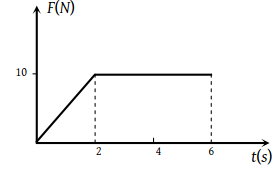(1) Zero

(2) 5 N-s

(3) 30 N-s

(4) 50 N-s

Concept Questions :-

Newton laws
High Yielding Test Series + Question Bank - NEET 2020

Difficulty Level:

The variation of momentum with time of one of the body in a two body collision is shown in fig. The instantaneous force is maximum corresponding to point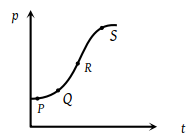(1) P

(2) Q

(3) R

(4) S

Concept Questions :-

Newton laws
High Yielding Test Series + Question Bank - NEET 2020

Difficulty Level:

Figures I, II, III and IV depict variation of force with time

 (I)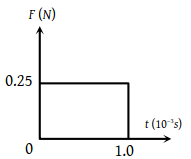(II)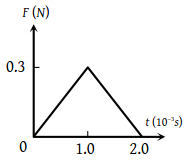(III)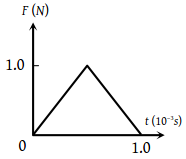(IV)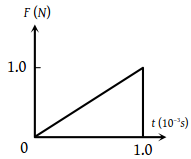The impulse is highest in the case of situations depicted. Figure

(1) I and II

(2) III and I

(3) III and IV

(4) IV only

Concept Questions :-

Newton laws
High Yielding Test Series + Question Bank - NEET 2020

Difficulty Level:

The masses of 10 kg and 20 kg respectively are connected by a massless spring as shown in figure. A force of 200 N acts on the 20 kg mass. At the instant shown, the 10 kg mass has acceleration 12 m/sec2. What is the acceleration of 20 kg mass?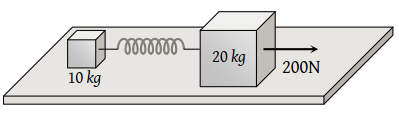(1) 12 m/sec2

(2) 4 m/sec2

(3) 10 m/sec2

(4) Zero

Concept Questions :-

Spring force
High Yielding Test Series + Question Bank - NEET 2020

Difficulty Level:

Two masses M and m are connected by a weightless string. They are pulled by a force F on a frictionless horizontal surface. The tension in the string will be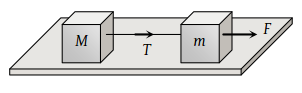(1) $\frac{FM}{m+M}$

(2) $\frac{F}{M+m}$

(3) $\frac{FM}{m}$

(4) $\frac{Fm}{M+m}$

Concept Questions :-

Types of forces
High Yielding Test Series + Question Bank - NEET 2020

Difficulty Level:

Two masses M and m are connected by a weightless string. They are pulled by a force F on a frictionless horizontal surface. The acceleration of mass m is(1) $\frac{F}{m}$

(2) $\frac{F-T}{m}$

(3) $\frac{F+T}{m}$

(4) $\frac{F}{M}$

Concept Questions :-

Types of forces
High Yielding Test Series + Question Bank - NEET 2020

Difficulty Level:

A uniform rope of length l lies on a table. If the coefficient of friction is μ, then the maximum length l1 of the part of this rope which can overhang from the edge of the table without sliding down is

(1) $\frac{l}{\mu }$

(2) $\frac{l}{\mu +l}$

(3) $\frac{\mu l}{1+\mu }$

(4) $\frac{\mu l}{\mu -1}$

Concept Questions :-

Friction
High Yielding Test Series + Question Bank - NEET 2020

Difficulty Level:

A heavy uniform chain lies on a horizontal table-top. If the coefficient of friction between the chain and table surface is 0.25, then the maximum fraction of length of the chain, that can hang over one edge of the table is

(1) 20%

(2) 25%

(3) 35%

(4) 15%

Concept Questions :-

Friction
High Yielding Test Series + Question Bank - NEET 2020

Difficulty Level:

The blocks A and B are arranged as shown in the figure. The pulley is frictionless. The mass of A is 10 kg. The coefficient of friction of A with the horizontal surface is 0.20. The minimum mass of B to start the motion will be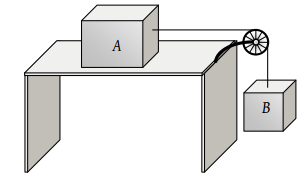(1) 2 kg

(2) 0.2 kg

(3) 5 kg

(4) 10 kg

Concept Questions :-

Types of forces